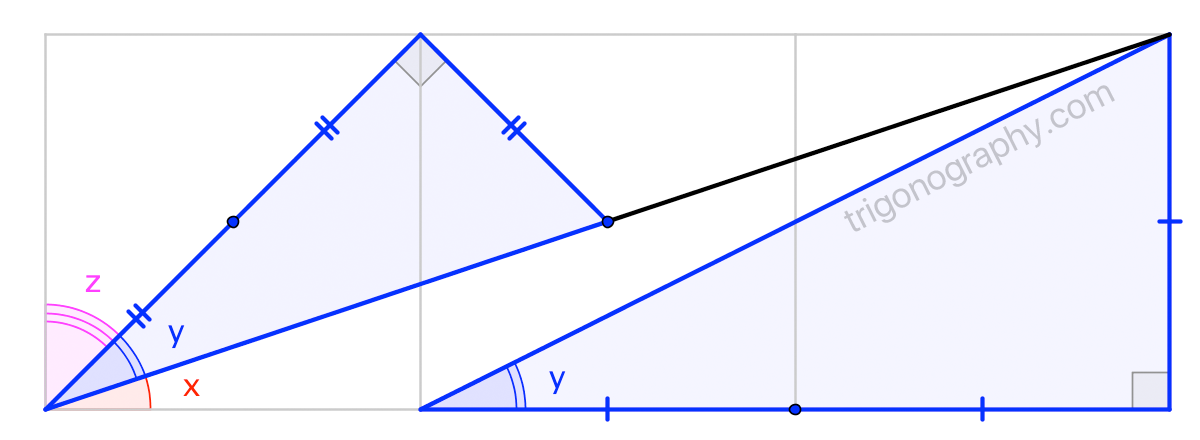## Three Squares Puzzle

$$\large x + y + z \;=\; \text{???}$$

A 1996 Math Horizons article by Martin Gardner ( re-printed here (PDF) ) posed this puzzle
and provided some references, including Charles Trigg(!)’s 1971 compilation of 54 solutions.

The following trigonograph is likely one of the Trigg’s 54; indeed, I see that the key observation
appears in the solution given by Gardner. (Even so, I think this approach is a bit more direct,
and the presentation, if I do say so myself, slightly “AHA!”-ier).

SPOILERS$$\large x + y + z \;=\; 90^\circ$$

Note. If you had a decent guess at the answer, then there’s an “obvious” proof using a trig formula.
(What is it?) But … formulas without pictures … What’s the fun in that?